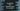# How to find the absolute value of a number in JavaScript## How to find the absolute value of a number in JavaScript:

JavaScript provides one method defined in the Math object that can be used to find the absolute value of a given number. It is abs(). In this post, we will learn how to use abs() function with examples.

## Definition of abs():

abs() method is defined as below:

``Math.abs(number);``

### Parameter:

It takes one parameter, the number to convert to an absolute value.

### Return value of abs():

abs() function returns the absolute value of the number we are passing. It returns NaN if the parameter is not a number.

This is defined in the Math object which contains this function.

## Example of abs():

Let’s take a look at abs() with different types of parameters:

``````console.log(Math.abs(10))
console.log(Math.abs(-10))
console.log(Math.abs(10.5))
console.log(Math.abs(-10.5))
console.log(Math.abs(0))``````

It will print:

``````10
10
10.5
10.5
0``````

### Example of abs that prints NaN:

``````console.log(Math.abs(undefined))
console.log(Math.abs('hello'))
console.log(Math.abs('&'))``````

It will print NaN for all.

``````NaN
NaN
NaN``````